# NHÀ khoa học nguyễn văN ĐẠo với lý thuyết dao đỘng và chuyểN ĐỘng hỗN ĐỘN

tải về 3.08 Mb.
 trang 9/11 Chuyển đổi dữ liệu 15.11.2017 Kích 3.08 Mb.
2.3 The forcing amplitude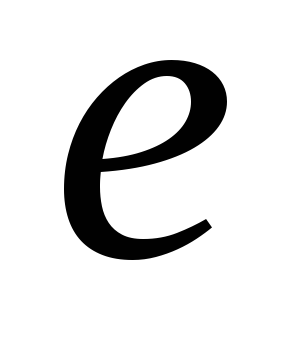is the control parameter

W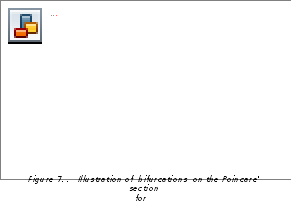e examine a graph of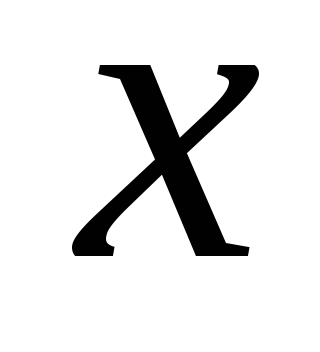versus the forcing amplitudeat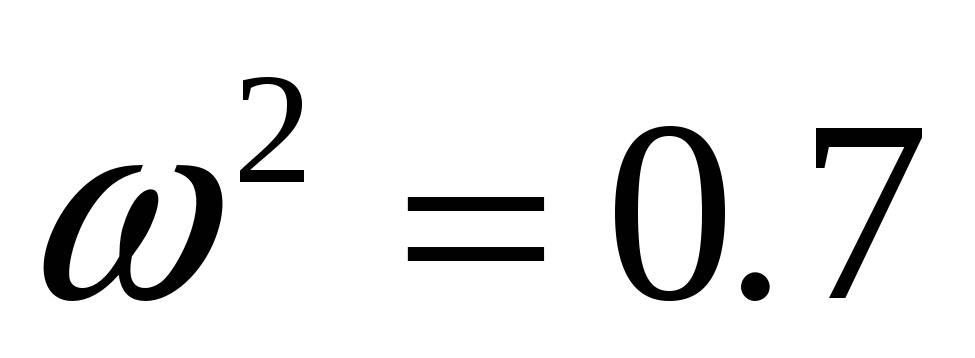,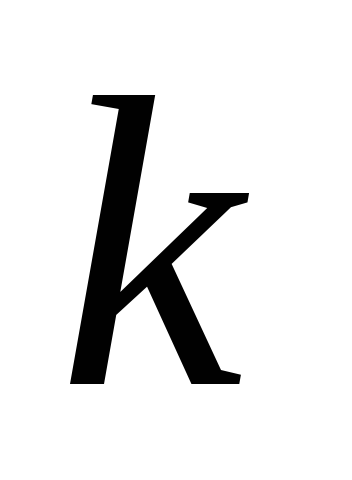=1 ,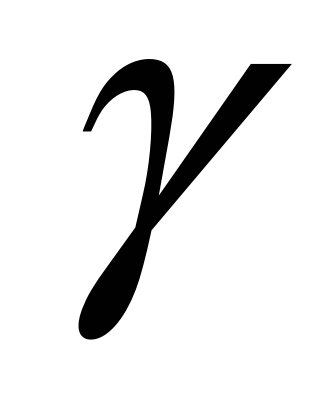=0.6 ,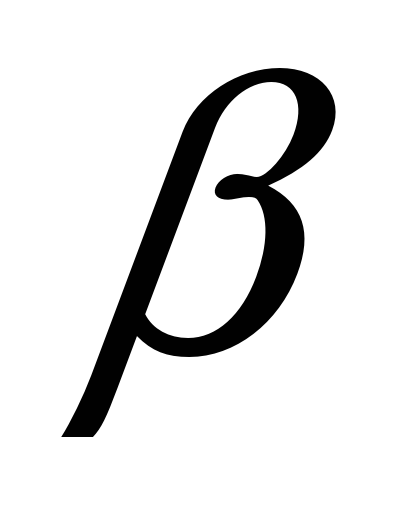= -1,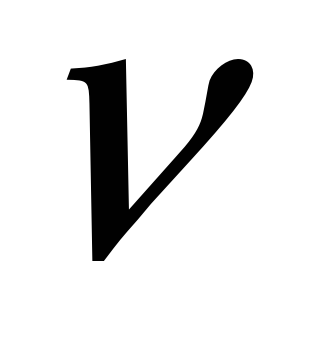= 0.837 in order to detect bifurcations. The bifurcation diagram is shown in Figure 7. In this numerically constructed bifurcation diagram, the discrete points on the Poincare’ section of the attractor realized at each value e are displayed.

Obviously, from Figure 7, we can observe the sequence of period-doubling bifurcations. First, with one of valuesin the interval (4.7 , 4.7645125), the Poincare’ section consists of five points (five dark points in Fig.8), so there exist the subharmonic motions with the period equaling five times of the period of the external force (Figure 8). At the value 4.7645125, the first period-doubling bifurcation occurs. After the bifurcation, with the valueswhich is in the right vicinity of the value 4.7645125, the subharmonic motions with the period equaling twice the period of the previous motions appear, the Poincare’ sections consist of ten points (Fig. 9), and so on. The chaotic attractor realized at e = 4.8042 appearing after a sequence of period-doubling bifurcations is shown in Figure 10, and Figure 11 is corresponding attractor. The largest Liapunov exponent evaluated is positive (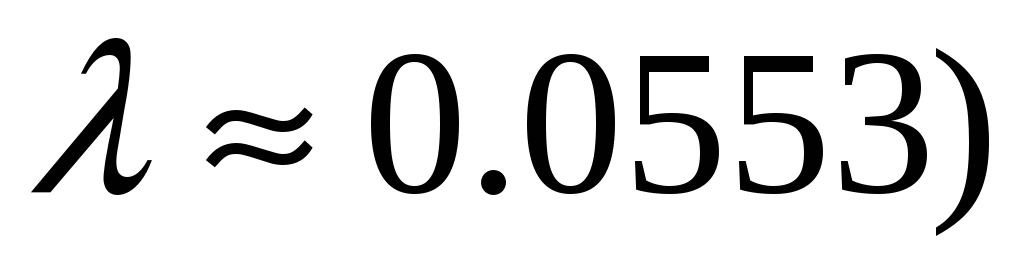defines sensitivity to initial conditions on the chaotic attractor.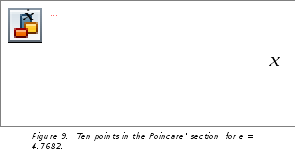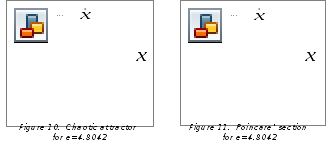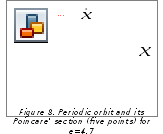The sequence of period-doubling bifurcations is one route to chaos and it is common

in many dynamical systems. It is particularly interesting because it may be characterized by a certain universal constant (called the Feigenbaum’s constant) that do not depend on nature of the concrete systems. This constant is considered as a specify criterion to determine if a system becomes chaotic by observing the prechaotic periodic behavior.

If the first bifurcation occurs at parameter value e1, the second at e2, and so on, then this constant is defined as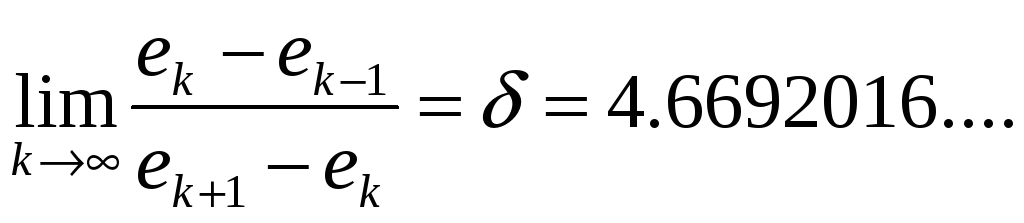Table 12 shows a list of the parameters at which period-doublings occur in the Poincare’ map corresponding to the system (1) for,=1 ,=0.6 ,= -1,= 0.837 and e is used as the control parameter.
 Period Parameter e Ratio 5 10 20 40 80 160 . 4.7645125 4.7717130 4.77327499 4.773611 4.77368297 . . 4.600144 4.656677 4.668751 . .

Cơ sở dữ liệu được bảo vệ bởi bản quyền ©hocday.com 2019
được sử dụng cho việc quản lý

Quê hương